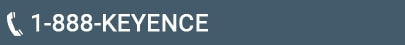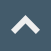# Basics of Dimension Inspection

## Dimensions/Edge Detection

Dimension measurement using edge detection is a recent trend of image sensor applications. In dimension inspection using image sensor, position, width, angle can be measured by capturing the object in two dimensions and detecting the edge. Here, the principle of edge detection is explained according to the processing process.
Understanding the principle makes it possible to set the detection to the optimum state. In addition, we introduce representative inspection examples using edges and explain how to select preprocessing filters for detection stabilization.

### Principle of Edge Detection

An edge is a border that separates a bright area from a dark area within an image. To detect an edge this border of different shades must be processed. Edges can be obtained through the following four process steps.

#### (1)Perform projection processing

Projection processing scans the image vertically to obtain the average intensity of each projection line. The average intensity waveform of each line is called the projected waveform.

##### What is the projection processing?

Projection processing is used to obtain the average intensity and reduce false detection caused by noise within the measurement area.

#### (2)Perform Differential Processing

Larger deviation values are obtained when the difference in shades are more distinct.

##### What is the differential processing?

Differential processing eliminates the influence caused by changes in absolute intensity values within the measurement area.

(Example) The absolute intensity value is "0" if there are no changes in shade. If color changes from white (255) to black (0), the variation is -255.

#### (3)Maximum Deviation Value Always Needs to be 100%

To stabilize the edge in actual production scenarios, internal compensation is performed so that the maximum deviation value is always maintained at 100%. Then, the edge position is determined from the peak point of the differential waveform where it exceeds the preset edge sensitivity (%). This method of edge normalization ensures that the edge's peak point is always detected, stabilizing image inspections that are prone to frequent changes in illumination.

#### (4)Perform Sub-Pixel Processing

Focus on the adjacent three pixels of the maximum differential waveform and perform interpolation calculations. Measure the edge position in units down to 1/100 of a pixel (sub-pixel processing).

### Examples of inspection using edge detection

Edge detection includes many of the tools shown below. This section introduces some examples of frequently used tools.

#### Example 1. Inspections using the edge position

By setting an edge position window at several places, the X and Y coordinates of the target object are measured.

#### Example 2. Inspections using the edge width tool

By using the “outer diameter” feature of the edge width tool, the width of the metal plate and the diameter of the hole in the X and Y directions can be measured.

#### Example 3. Inspections using the circumference area of the profile position

By setting the measurement area as “circumference,” the angle (phase) of the notch is measured.

#### Example 4. Inspections using the profile width

Use the "trend edge width" tool to scan the internal diameter and evaluate the degree of flatness.

#### PROFILE POSITION TOOL

The profile position tool combines a group of narrow edge windows to detect the edge position of each point. Since all of the data is collected within one inspection tool, it becomes easy to detect minute fluctuations by calculating minimum, maximum, and average values over the entire part.

##### Detection principle

By moving the narrow area segments in small pitches, the edge width and edge position of each point is detected.

If highly accurate position detection is required,
Reduce the segment size.
If highly accurate position detection is required,
Reduce the shift width of the segment.
If highly accurate position detection is required,
The direction towards which the segment is moved.

### Pre-processing filter to further stabilize edge detection

In edge detection, it is very important to suppress the variations of edges. "Median" and "average" filters are effective at stabilizing edge detections. This section explains the characteristics of these pre-processing filters and effective selection method.

#### Original image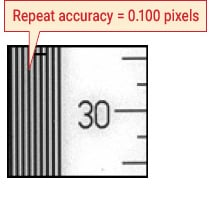#### Averaging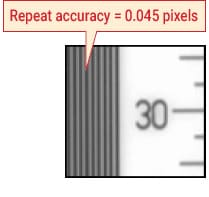Averaging filter with 3 × 3 pixels. This filter is effective in reducing the influence of noise components.

#### Median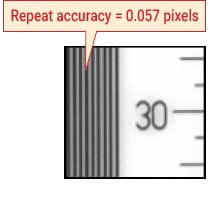Median filter with 3×3 pixels. This filter reduces the influence of noise components without blurring the image edge.

#### How to optimize the pre-processing filter

Though “median” and “averaging” generally lead to the stabilization of edges, it is difficult to know which is effective for the target object. This section introduces a method of statistically evaluating the variations of measurements when these filters are used.

The CV-X series (CV2000 or later) is equipped with a statistical analysis function. This function records the measured data internally and performs statistical analysis simultaneously.

By repetitively measuring the static target with “no filter,” “median,” “averaging,” “median + averaging,” and “averaging + medial” the optimum filter can be selected.

### Summary

Note the following four points to effectively utilize edge tools with an image sensor:

• By understanding the edge detection principle, proper adjustments can be made with ease.
• By understanding the capabilities of different edge tools the possibility of accurate inspection is significantly improved.
• By referencing typical detection examples, accurate detection can be implemented quickly.
• By selecting an optimum pre-processing filter, detection can be stabilized.

The next topic is the principle and applications of position measurement (search mode). Let's look at the principle and applications of search mode, which is not only used for the positioning of parts in assembly processes, but also used as a position correction function.